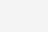# 计算机进制位转换

2进制，用两个阿拉伯数字：0、1；

8进制，用八个阿拉伯数字：0、1、2、3、4、5、6、7；

10进制，用十个阿拉伯数字：0到9；

16进制就是逢16进1，但我们只有0~9这十个数字，所以我们用A，B，C，D，E，F这五个字母来分别表示10，11，12，13，14，15。字母不区分大小写。

1101100 ←二进制数

6543210 ←排位方法

1*26 + 1*25 + 0*24 + 1*23 + 1* 22 + 0*21 + 0*20

↑            ↑

=64+32+0+8+4+0+0

=108

110 = 4+2 = 6

111 = 4+2+1 = 7

011 = 2+1 = 3

0101 1011 1011

0101 = 4+1 = 5

1011 = 8+2+1 = 11（由于10为A，所以11即B）

1011 = 8+2+1 = 11（由于10为A，所以11即B）

(别忘了，在上面的计算中，A表示10，而F表示15)、(0)

4250

5290

7991

5720

7330

5850

6270

6800

4270

4910